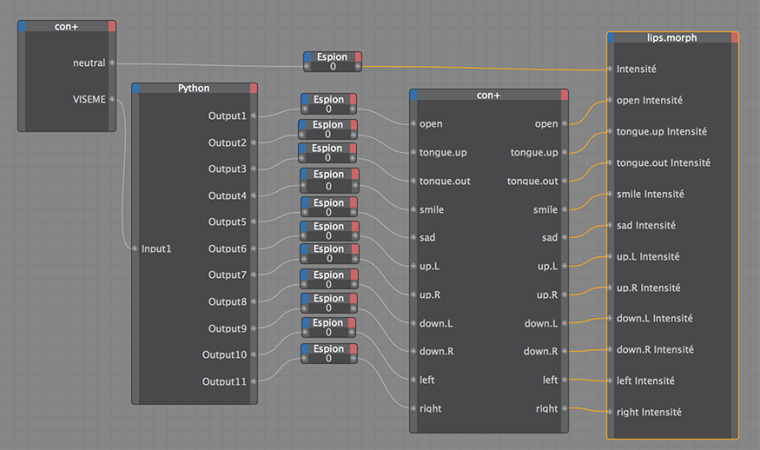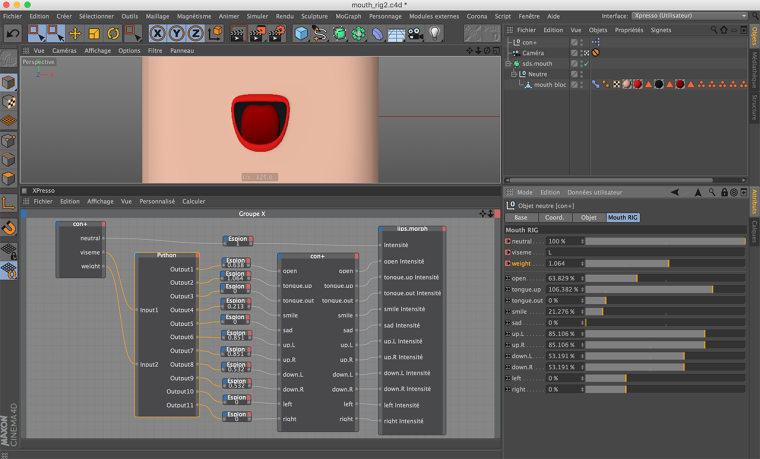# Viseme RIG test

Hello,

in order to faster the mouth animation process of a character,
i try to create a viseme rig.

I have put on an object a pose morph tag with 6 sliders for 6 visemes.
I created a dynamic text in user data, to edit viseme orders .
I created an Xpresso tag, with python node, to control visemes sliders.
It works, but in a boolean way !

I want to improve this Xpresso and python Rig to control sliders
in a linear way (from 0% to 100%, or 100% to 0%) !
With duration for the moving sliders between viseme orders
With conditions for the position of the sliders (e.g.: if slider 1 at position 0, go to 100, if slider 2 at position 100, go to 0 ....).

How can i do it ?
I'm a python rookie !

Thank's

Hi Gal, thanks for writing us.

With regard to the issue reported, it looks to me like some sorting of unwanted data-clamping effect due to type casting. To be more helpful please provide your code snippet ( or a complete test asset) in order to dig further.

Best, Riccardo

@r_gigante said in Viseme RIG test:

With regard to the issue reported, it looks to me like some sorting of unwanted data-clamping effect due to type casting. To be more helpful please provide your code snippet ( or a complete test asset) in order to dig further.

Hi Ricardo,

I've a little bit improved my xpresso nodes, simple but efficient !The viseme are controlled in a boolean way = step key frame.
With controlling the global intensity of the pose morph tag,
i can have more interpolation of the lips movement between the viseme step keys frames.
Mouth rig test_animatic

How to improved python code in order to have viseme linear key frames ?

Thanks
Best regards
Gal

This is my simple python code :

``````import c4d
#Welcome to the world of Python

def main():
global Output1, Output2, Output3, Output4,  Output5,  Output6,  Output7,  Output8,  Output9,  Output10,  Output11

if Input1 == "":
Output1 = 0
Output2 = 0
Output3 = 0
Output4 = 0
Output5 = 0
Output6 = 0
Output7 = 0
Output8 = 0
Output9 = 0
Output10 = 0
Output11 = 0

if Input1 == "E":
Output1 = 1
Output2 = 0
Output3 = 0
Output4 = 0
Output5 = 0
Output6 = 0
Output7 = 0
Output8 = 0
Output9 = 0
Output10 = 0
Output11 = 0

if Input1 == "A":
Output1 = 0.8
Output2 = 0
Output3 = 0
Output4 = 0.8
Output5 = 0
Output6 = 0
Output7 = 0
Output8 = 0
Output9 = 0
Output10 = 0.5
Output11 = 0.5

if Input1 == "I":
Output1 = 0
Output2 = 0
Output3 = 0
Output4 = 1
Output5 = 0
Output6 = 1
Output7 = 1
Output8 = 0
Output9 = 0
Output10 = 0.5
Output11 = 0.5

if Input1 == "U":
Output1 = 0.8
Output2 = 0
Output3 = 0
Output4 = 0
Output5 = 0
Output6 = 0
Output7 = 0
Output8 = 1
Output9 = 1
Output10 = 0
Output11 = 0

if Input1 == "R":
Output1 = 0.5
Output2 = 0
Output3 = 0
Output4 = 1
Output5 = 0
Output6 = -0.5
Output7 = -0.5
Output8 = 1
Output9 = 1
Output10 = 0.5
Output11 = 0.5

if Input1 == "O":
Output1 = 0.9
Output2 = 0
Output3 = 0
Output4 = 0
Output5 = 0
Output6 = 0
Output7 = 0
Output8 = 0.4
Output9 = 0.4
Output10 = -0.3
Output11 = -0.3

if Input1 == "CH":
Output1 = 0.2
Output2 = 0
Output3 = 0
Output4 = 0.35
Output5 = 0
Output6 = 0.5
Output7 = 0.5
Output8 = 0
Output9 = 0
Output10 = 0.6
Output11 = 0.6

if Input1 == "S":
Output1 = 0.1
Output2 = 0
Output3 = 0
Output4 = 0.41
Output5 = 0.2
Output6 = 1
Output7 = 1
Output8 = 1
Output9 = 1
Output10 = 1
Output11 = 1

if Input1 == "M":
Output1 = 0
Output2 = 0
Output3 = 0
Output4 = 0.1
Output5 = 0.3
Output6 = 0.6
Output7 = 0.6
Output8 = 0.85
Output9 = 0.85
Output10 = 0.4
Output11 = 0.4

if Input1 == "D":
Output1 = 0.05
Output2 = 0
Output3 = 0
Output4 = 0.3
Output5 = 0.2
Output6 = 0.8
Output7 = 0.8
Output8 = 0.85
Output9 = 0.85
Output10 = 0.2
Output11 = 0.2

if Input1 == "F":
Output1 = 0.05
Output2 = 0
Output3 = 0
Output4 = 0
Output5 = 0.2
Output6 = 0.8
Output7 = 0.8
Output8 = 0.5
Output9 = 0.5
Output10 = 0
Output11 = 0

if Input1 == "L":
Output1 = 0.6
Output2 = 1
Output3 = 0
Output4 = 0.2
Output5 = 0
Output6 = 0.8
Output7 = 0.8
Output8 = 0.5
Output9 = 0.5
Output10 = 0
Output11 = 0``````

Hi Gal, thanks for following-up.

With regard to your setup, without changing too much things, you could simply consider to add an additional float parameter to your VISEME data - in order to have three input params "neutral", "VISEME" and a "weight" - and use its value defined by a curve in your python script (below to keep your naming scheme is called Input2) in order to change the weight of your VISEME rig.

``````import c4d

def main():

global Output1, Output2, Output3, Output4,  Output5,  Output6,  Output7,  Output8,  Output9,  Output10,  Output11

# Input2 is the float parameter defined to weight the viseme
Output1 = 0
Output2 = 0
Output3 = 0
Output4 = 0
Output5 = 0
Output6 = 0
Output7 = 0
Output8 = 0
Output9 = 0
Output10 = 0
Output11 = 0

if Input1 == "E":
Output1 = 1.0 * Input2
elif Input1 == "A":
Output1 = 0.8 * Input2
Output4 = 0.8 * Input2
Output10 = 0.5 * Input2
Output11 = 0.5 * Input2
elif Input1 == "I":
Output4 = 1.0 * Input2
Output6 = 1.0 * Input2
Output7 = 1.0 * Input2
Output10 = 0.5 * Input2
Output11 = 0.5 * Input2
elif Input1 == "U":
Output1 = 0.8 * Input2
Output8 = 1.0 * Input2
Output9 = 1.0 * Input2
elif Input1 == "R":
Output1 = 0.5 * Input2
Output4 = 1.0 * Input2
Output6 = -0.5 * Input2
Output7 = -0.5 * Input2
Output8 = 1.0 * Input2
Output9 = 1.0 * Input2
Output10 = 0.5 * Input2
Output11 = 0.5 * Input2
elif Input1 == "O":
Output1 = 0.9 * Input2
Output8 = 0.4 * Input2
Output9 = 0.4 * Input2
Output10 = -0.3 * Input2
Output11 = -0.3 * Input2
elif Input1 == "CH":
Output1 = 0.2 * Input2
Output4 = 0.35 * Input2
Output6 = 0.5 * Input2
Output7 = 0.5 * Input2
Output10 = 0.6 * Input2
Output11 = 0.6 * Input2
elif Input1 == "S":
Output1 = 0.1 * Input2
Output4 = 0.41 * Input2
Output5 = 0.2 * Input2
Output6 = 1.0 * Input2
Output7 = 1.0 * Input2
Output8 = 1.0 * Input2
Output9 = 1.0 * Input2
Output10 = 1.0 * Input2
Output11 = 1.0 * Input2
elif Input1 == "M":
Output4 = 0.1 * Input2
Output5 = 0.3 * Input2
Output6 = 0.6 * Input2
Output7 = 0.6 * Input2
Output8 = 0.85 * Input2
Output9 = 0.85 * Input2
Output10 = 0.4 * Input2
Output11 = 0.4 * Input2
elif Input1 == "D":
Output1 = 0.05 * Input2
Output4 = 0.3 * Input2
Output5 = 0.2 * Input2
Output6 = 0.8 * Input2
Output7 = 0.8 * Input2
Output8 = 0.85 * Input2
Output9 = 0.85 * Input2
Output10 = 0.2 * Input2
Output11 = 0.2 * Input2
elif Input1 == "F":
Output1 = 0.05 * Input2
Output5 = 0.2 * Input2
Output6 = 0.8 * Input2
Output7 = 0.8 * Input2
Output8 = 0.5 * Input2
Output9 = 0.5 * Input2
elif Input1 == "L":
Output1 = 0.6 * Input2
Output2 = 1.0 * Input2
Output4 = 0.2 * Input2
Output6 = 0.8 * Input2
Output7 = 0.8 * Input2
Output8 = 0.5 * Input2
Output9 = 0.5 * Input2
``````

Hoping it make sense, feel free to get back for any further help.

Cheer, Riccardo

Hello

Thank you Ricardo for the code and this option.
It works for me !Is there a way to control an interpolation of data (e.g. 0 to 100) on a duration (e.g. 10 frames) with python, with only one order keyframe ?

If you have an advice or a weblink to better learn python for C4D, i'll take it !

Thanks

Gal

Hi! You could go for CTracks via Python. You'd have to get the track for a position parameter, for example, or create one, then get curve, then add keys. Later, you could simply range map it back to your Xpresso rig. it It's definitely doable!